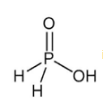Courses

# Test: Level - 1 P - Block Elements - 1

## 25 Questions MCQ Test Chemistry Class 12 | Test: Level - 1 P - Block Elements - 1

Description
This mock test of Test: Level - 1 P - Block Elements - 1 for Class 12 helps you for every Class 12 entrance exam. This contains 25 Multiple Choice Questions for Class 12 Test: Level - 1 P - Block Elements - 1 (mcq) to study with solutions a complete question bank. The solved questions answers in this Test: Level - 1 P - Block Elements - 1 quiz give you a good mix of easy questions and tough questions. Class 12 students definitely take this Test: Level - 1 P - Block Elements - 1 exercise for a better result in the exam. You can find other Test: Level - 1 P - Block Elements - 1 extra questions, long questions & short questions for Class 12 on EduRev as well by searching above.
QUESTION: 1

### Which among the following compound, nitrogen shows the oxidation state of +5?

Solution:

N has +5 in HNO3. Oxidation state of O is -2 and H is +1.​

QUESTION: 2

### Which among the following forms basic oxide?

Solution:

As we move down the group metallic character increases so Bi is a metal thus its oxide is basic.

QUESTION: 3

### In laboratory ammonia is prepared by heating

Solution:

2(NH4Cl) + Ca(OH)2 → (CaCl)2 + 2(NH3) + 2(H2O)
Hence C is correct.

QUESTION: 4

Ammonia has a higher boiling point and is less volatile because of

Solution:

NHundergo intermolecular hydrogen bonding and therefore it has higher boiling point compared to other hydrides of group 15.

QUESTION: 5

Which compound is used as the cooling liquid in refrigerators?

Solution:

NH3  is used as cooling liquid in refrigerators.

QUESTION: 6

At what temperature white phosphorous changes to red phosphorous?

Solution:

White phosphorus when heated to 300° C polymerization occurs and it changes to Red Phosphorus.

QUESTION: 7is the structure of

Solution:

H3PO is Hypophosphorus acid

QUESTION: 8

Elements of which group are known as ore forming elements?

Solution:

Maximum ores are of group 16 elemnts (O, S etc).

QUESTION: 9

Most metal oxides are

Solution:

Metal oxides are basic and ionic in nature.

QUESTION: 10

The stability of hydrides of group 16 elements decreases in the order

Solution:

As we move down the group bond length increases therefore stability of hydrides decreases. Order of bond length O-H < S-H < Se-H < Te-H.

QUESTION: 11

Formula of oleum is

Solution:

Oleum is H2S2O7

QUESTION: 12

Which among the following does not exhibit positive oxidation state?

Solution:

Fluorine is most electronegative element therefore can only gain electrons. Thus it only shows negative oxidation state.

QUESTION: 13

Which of the following is the strongest Lewis base?

Solution:

Because of lower electronegativity of I

QUESTION: 14

Hydrogen sulphide reacts with lead acetate forming a black compound which reacts with hydrogen peroxide to form another compound. The colour of the compound is

Solution:

PbS + H2O2 → PbSo4(white) + H2O

QUESTION: 15

Which of the following is thermally the most stable?

Solution:

Stability of hydrides decreases down the group so most stable is H2O

QUESTION: 16

Which of the following is prepared by cyanamide process?

Solution:

CaNCN + 3H2O → CaCO+ 2NH3

QUESTION: 17

Ozone can be detected by using

Solution:

Ozone is detected by using Hg.

QUESTION: 18

Which of the following oxides reacts with both HCl and NaOH?

Solution:

ZnO is an amphroteric oxide.

QUESTION: 19

Name the gas whose formula was established by Sorret?

Solution:

Sorret established formula of ozone.

QUESTION: 20

Which colour is given by CuSO4 with ammonia?

Solution:

Cu2+  + 4NH3 → [Cu (NH3)4]2+ (blue)

QUESTION: 21

Xenondifluoride is

Solution:

CN = 0.5(V+M-C+A) For XeF2 CN = 5 so shape will be linear and structure will be trigonal pyramidal.

QUESTION: 22

A metal X on heating in nitrogen gas gives Y. Y on treatment with water gives a colourless gas which when passed through copper sulphate solution gives a blue colour. Y is

Solution:

Mg3Nreacts with water to evolve NH3. Which reacts with copper ion to form blue color complex.

QUESTION: 23

The reagent used to distinguish between H2O2 and Ois:

Solution:

H2Ocan decolorize the color of KMnO4

QUESTION: 24

When chlorine is passed through concentrated hot solution of KOH, the compound formed is

Solution:

Clon treatment with conc. Base form ClO3-

QUESTION: 25

Ammonia can be dried using

Solution:

CaO is used to dry NH3